Succeed with maths – Part 1

Start this free course now. Just create an account and sign in. Enrol and complete the course for a free statement of participation or digital badge if available.

Free course

3 Division

You know from the last section that multiplication is repeated addition, similarly division can be thought of as repeated subtraction. For example, 20 divided by 4 is 5 (20 ÷ 4 = 5). The answer is 5 because 5 is the number of times you subtract 4 from 20 to arrive at zero: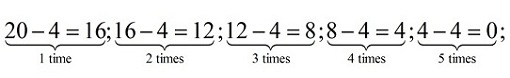Figure 6 Demonstrating division

This also leads to the realisation that division is the opposite or undoes multiplication. If you add 4, five times to zero (repeated addition or multiplication) then you will arrive back at 20.

And just as with the other operators, there are words that tell us you need to divide.

Here are some examples that you may recognise:

• How many times does 8 go into 72?
• How can you share 72 items equally among eight people?
• How many lots of five are there in 20?

Unlike multiplication when there is only one symbol that says multiply, division has a whole family of notation! If you want to divide 72 by 8 this can be written in the following ways:

72 ÷ 8, or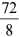, or 72/8 or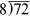Not all division problems will result in a nice whole number answer and you may have a number left over. This number is known as the remainder. The common notation is to show this using the letter R.

Now, you’re going to look at division on paper, just as you did with multiplication.

You set up division on paper using the final notation from our list. Starting from the left, in this case, divide into each number in turn, writing the result above the number you have divided into. In this case if you can’t divide into a number move to the next and divide into both.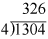Before the next activity you might like to watch this video showing you how to carry out long division.

Skip transcript

Transcript

Narrator
Let's now see if we can divide into larger numbers. And just as a starting point, in order to divide into larger numbers, you at least need to know your multiplication tables from the 1–multiplication tables all the way to, at least, the 10–multiplication.So all the way up to 10 times 10, which you know is 100.
And then, starting at 1 times 1 and going up to 2 times 3, all the way up to 10 times 10.And, at least when I was in school, we learned through 12 times 12. But 10 times 10 will probably do the trick. And that's really just the starting point. Because to do multiplication problems like this, for example, or division problems like this.
Let's say I'm taking 25 and I want to divide it by 5. So I could draw 25 objects and then divide them into groups of 5 or divide them into 5 groups and see how many elements are in each group. But the quick way to do is just to think about, well 5 times what is 25, right? 5 times question mark is equal to 25. And if you know your multiplication tables, especially your 5-multiplication tables, you know that 5 times 5 is equal to 25. So something like this, you'll immediately just be able to say, because of your knowledge of multiplication, that 5 goes into 25 five times.
And you'd write the 5 right there. Not over the 2, because you still want to be careful of the place notation. You want to write the 5 in the ones place. It goes into t 5 ones times, or exactly five times. And the same thing. If I said 7 goes into 49. That's how many times? Well you say, that's like saying 7 times what-- you could even, instead of a question mark, you could put a blank there --7 times what is equal to 49? And if you know your multiplication tables, you know that 7 times 7 is equal to 49.
All the examples I've done so far is a number multiplied by itself. Let me do another ample. Let me do 9 goes into 54 how many times? Once again, you need to know your multiplication tables to do this. 9 times what is equal to 54? And sometimes, even if you don't have it memorized, you could say 9 times 5 is 45. And 9 times 6 would be 9 more than that, so that would be 54.
So 9 goes into 54 six times. So just as a starting point, you need to have your multiplication tables from 1 times 1 all the way up the 10 times 10 memorized. In order to be able to do at least some of these more basic problems relatively quickly. Now, with that out of the way, let's try to do some problems that's might not fit completely cleanly into your multiplication tables. So let's say I want to divide-- I am looking to divide 3 into 43. And, once again, this is larger than 3 times 10 or 3 times 12.
Actually, look. Well, let me do another problem. Let me do 3 into 23. And, if you know your 3-times tables, you realize that there's 3 times nothing is exactly 23. I'll do it right now. 3 times 1 is 3. 3 times 2 is 6. Let me just write them all out. 3 times 3 is 9, 12, 15, 18, 21, 24, right?
There's no 23 in the multiples of 3. So how do you do this division problem? Well what you do is you think of what is the largest multiple of 3 that does go into 23? And that's 21. And 3 goes into 21 how many times? Well you know that 3 times 7 is equal to 21. So you say, well 3 will go into 23 seven times. But it doesn't go into it cleanly because 7 times 3 is 21. So there's a remainder left over. So if you take 23 minus 21, you have a remainder of 2. So you could write that 23 divided by 3 is equal to 7 remainder-- maybe I'll just, well, write the whole word out --remainder 2.
So it doesn't have to go in completely cleanly. And, in the future, we'll learn about decimals and fractions. But for now, you just say, well it goes in cleanly 7 times, but that only gets us to 21. But then there's 2 left over. So you can even work with the division problems where it's not exactly a multiple of the number that you're dividing into the larger number. But let's do some practice with even larger numbers. And I think you'll see a pattern here. So let's do 4 going into-- I'm going to pick a pretty large number here --344. And, immediately when you see that you might say, hey Sal, I know up to 4 times 10 or 4 times 12. 4 times 12 is 48. This is a much larger number. This is way out of bounds of what I know in my 4-multiplication tables. And what I'm going to show you right now is a way of doing this just knowing your 4 multiplication tables. So what you do is you say 4 goes into this 3 how many times?
And you're actually saying 4 goes into this 3 how many hundred times? So this is-Because this is 300, right? This is 344. But 4 goes into 3 no hundred times, or 4 goes into-- I guess the best way to think of it --4 goes 3 0 times. So you can just move on. 4 goes into 34. So now we're going to focus on the 34. So 4 goes into 34 how many times?
And here we can use our 4-multiplication tables. 4-- Let's see, 4 times 8 is equal to 32. 4 times 9 is equal to 36. So 4 goes into 34-- 30-- 9 is too many times, right? 36 is larger than 34. So 4 goes into 34 eight times. There's going to be a little bit left over. 4 goes in the 34 eights times. So let's figure out what's left over. And really we're saying 4 goes into 340 how many ten times? We're actually saying 4 goes into 340 eighty times. Because notice we wrote this 8 in the tens place. But just for our ability to do this problem quickly, you just say 4 goes into 34 eight times, but make sure you write the 8 in the tens place right there. 8 times 4. We already know what that is.
8 times 4 is 32. And then we figure out the remainder. 34 minus 32. Well, 4 minus 2 is 2. And then these 3's cancel out. So you're just left with a 2. But notice we're in the tens column, right? This whole column right here, that's the tens column. So really what we said is 4 goes into 340 eighty times. 80 times 4 is 320, right? Because I wrote the 3 in the hundreds column. And then there is-- and I don't want to make this look like a-- I don't want to make this look like a-- Let me clean this up a little bit. I didn't want to make that line there look like a-- when I was dividing the columns --to look like a 1. But then there's a remainder of 2, but I wrote the tens place. So it's actually a remainder of 20. But let me bring down this 4. Because I didn't want to just divide into 340. I divided into 344. So you bring down the 4. Let me switch colors. And then-- So another way to think about it. We just said that 4 goes into 344 eighty times, right? We wrote the 8 in the tens place. And then 8 times 4 is 320.
The remainder is now 24. So how many times does 4 go into 24? Well we know that. 4 yimes 6 is equal to 24. So 4 goes into 24 six times. And we put that in the ones place. 6 times 4 is 24. And then we subtract. 24 minus 24. That's-- We subtract at that stage, either case. And we get 0. So there's no remainder. So 4 goes into 344 exactly eighty-six times. So if your took 344 objects and divided them into groups of 4, you would get 86 groups. Or if you divided them into groups of 86, you would get 4 groups. Let's do a couple more problems. I think you're getting the hang of it. Let me do 7-- I'll do a simple one. 7 goes into 91. So once again, well, this is beyond 7 times 12, which is 84, which you know from our multiplication tables. So we use the same system we did in the last problem. 7 goes into 9 how many times? 7 goes into 9 one time. 1 times 7 is 7. And you have 9 minus 7 is 2. And then you bring down the 1. 21. And remember, this might seem like magic, but what we really said was 7 goes into 90 ten times-- 10 because we wrote the 1 in the tens place --10 times 7 is 70, right?
You can almost put a 0 there if you like. And 90 with the remainder-- And 91 minus 70 is 21. goes into 91 ten times remainder 21. And then you say 7 goes into 21-- Well you know that. 7 times 3 is 21. So 7 goes into 21 three times. 3 times 7 is 21. You subtract these from each other. Remainder 0. So 91 divided by 7 is equal to 13. Let's do another one. And I won't take that little break to explain the places and all of that. I think you understand that. I want, at least, you to get the process down really really well in this video. So let's do 7-- I keep using the number 7. Let me do a different number. Let me do 8 goes into 608 how many times? So I go 8 goes into 6 how many times? It goes into it 0 time. So let me keep moving. 8 goes into 60 how many times? Let me write down the 8. Let me draw a line here so we don't get confused. Let me scroll down a little bit. I need some space above the number. So 8 goes into 60 how many times? We know that 8 times 7 is equal to 56. And that 8 times 8 is equal to 64. So 8 goes into-- 64 is too big. So it's not this one. So 8 goes into 60 seven times. And there's going to be a little bit left over. So 8 goes into 60 seven times. Since we're doing the whole 60, we put the 7 above the ones place in the 60, which is the tens place in the whole thing.
7 times 8, we know, is 56. 60 minus 56. That's 4. We could do that in our heads. Or if we wanted, we can borrow. That be a 10. That would be a 5. 10 minus 6 is 4. Then you bring down this 8. 8 goes into 48 how many times? Well what's 8 times 6? Well, 8 times 6 is exactly 48. So 8 times-- 8 goes into 48 six times. 6 times 8 is 48. And you subtract. We subtracted up here as well. 48 minus 48 is 0. So, once again, we get a remainder of 0. So hopefully, that gives you the hang of how to do these larger division problems. And all we really need to know to be able to do these, to tackle these, is our multiplication tables up to maybe 10 times 10 or 12 times 12.
End transcript

Interactive feature not available in single page view (see it in standard view).

Now have a go at the next activity. If you wish you can view the times table [Tip: hold Ctrl and click a link to open it in a new tab. (Hide tip)]   again.

Activity 4 Division

Timing: Allow approximately 10 minutes

On a piece of paper, perform the following operations by hand.

(a) 4968 ÷ 24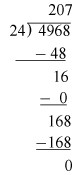The solution is 207.

(b) 4035 ÷ 15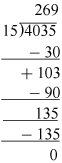The solution is 269.

When looking at subtraction and addition you thought about whether it mattered in what order these were carried out. You found that for addition the answer is the same whatever the order but this is not the case for subtraction. What about multiplication and division? You’ll find out in the next section.